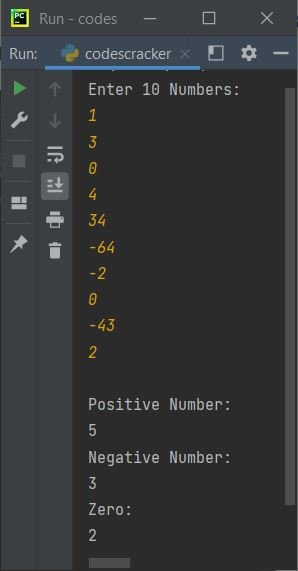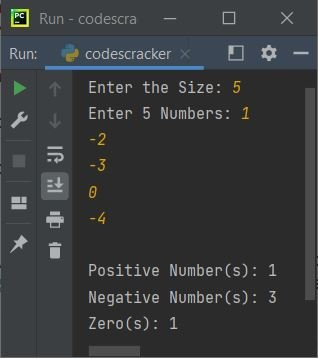# Python Program to Count Positive, Zero, Negative Numbers in List

• Count Positive, Zero, and Negative Numbers in a List of 10 Elements (Numbers)
• Count Positive, Zero, and Negative Numbers in a List of n Elements (Numbers)

## Count Positive, Zero, Negative Numbers in a List of 10 Elements

The question is, write a Python program to count total positive, zero, and negative numbers available in a given list. Here is its answer:

```nums = []
totPositive = 0
totNegative = 0
totZero = 0

print("Enter 10 Numbers: ")
for i in range(10):
nums.insert(i, int(input()))

for i in range(10):
if nums[i]>0:
totPositive = totPositive+1
elif nums[i]<0:
totNegative = totNegative+1
else:
totZero = totZero+1

print("\nPositive Number: ")
print(totPositive)
print("Negative Number: ")
print(totNegative)
print("Zero: ")
print(totZero)```

Here is its sample run:Now enter any 10 numbers as input say 1, 3, 0, 4, 34, -64, -2, 0, -43, 2 and press `ENTER` key to count and print total number of positive and negative numbers along with zeros:## Count Positive, Zero, Negative Numbers from a Series of n Numbers

This is little similar to previous program. The only main difference is, this program allows user to define the size of list too.

```nums = []
totPositive = 0
totNegative = 0
totZero = 0

print(end="Enter the Size: ")
s = int(input())

print(end="Enter " +str(s)+ " Numbers: ")
for i in range(s):
nums.insert(i, int(input()))

for i in range(s):
if nums[i]>0:
totPositive = totPositive+1
elif nums[i]<0:
totNegative = totNegative+1
else:
totZero = totZero+1

print(end="\nPositive Number(s): " +str(totPositive))
print("\nNegative Number(s): " +str(totNegative))
print("Zero(s): " +str(totZero))```

Here is its sample run with user input, 5 as size and 1, -2, -3, 0, -4 as five numbers:Python Online Test

« Previous Program Next Program »